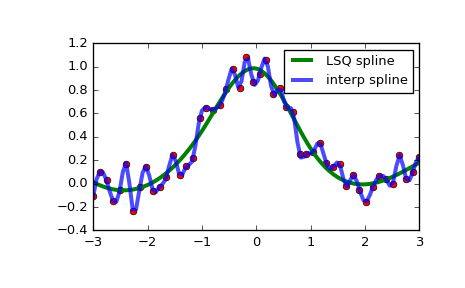# scipy.interpolate.make_lsq_spline¶

scipy.interpolate.make_lsq_spline(x, y, t, k=3, w=None, axis=0, check_finite=True)[source]

Compute the (coefficients of) an LSQ B-spline.

The result is a linear combination

$S(x) = \sum_j c_j B_j(x; t)$

of the B-spline basis elements, $$B_j(x; t)$$, which minimizes

$\sum_{j} \left( w_j \times (S(x_j) - y_j) \right)^2$
Parameters: x : array_like, shape (m,) Abscissas. y : array_like, shape (m, ...) Ordinates. t : array_like, shape (n + k + 1,). Knots. Knots and data points must satisfy Schoenberg-Whitney conditions. k : int, optional B-spline degree. Default is cubic, k=3. w : array_like, shape (n,), optional Weights for spline fitting. Must be positive. If None, then weights are all equal. Default is None. axis : int, optional Interpolation axis. Default is zero. check_finite : bool, optional Whether to check that the input arrays contain only finite numbers. Disabling may give a performance gain, but may result in problems (crashes, non-termination) if the inputs do contain infinities or NaNs. Default is True. b : a BSpline object of the degree k with knots t.

BSpline
base class representing the B-spline objects
make_interp_spline
a similar factory function for interpolating splines
LSQUnivariateSpline
a FITPACK-based spline fitting routine
splrep
a FITPACK-based fitting routine

Notes

The number of data points must be larger than the spline degree k.

Knots t must satisfy the Schoenberg-Whitney conditions, i.e., there must be a subset of data points x[j] such that t[j] < x[j] < t[j+k+1], for j=0, 1,...,n-k-2.

Examples

Generate some noisy data:

>>> x = np.linspace(-3, 3, 50)
>>> y = np.exp(-x**2) + 0.1 * np.random.randn(50)


Now fit a smoothing cubic spline with a pre-defined internal knots. Here we make the knot vector (k+1)-regular by adding boundary knots:

>>> from scipy.interpolate import make_lsq_spline, BSpline
>>> t = [-1, 0, 1]
>>> k = 3
>>> t = np.r_[(x,)*(k+1),
...           t,
...           (x[-1],)*(k+1)]
>>> spl = make_lsq_spline(x, y, t, k)


For comparison, we also construct an interpolating spline for the same set of data:

>>> from scipy.interpolate import make_interp_spline
>>> spl_i = make_interp_spline(x, y)


Plot both:

>>> import matplotlib.pyplot as plt
>>> xs = np.linspace(-3, 3, 100)
>>> plt.plot(x, y, 'ro', ms=5)
>>> plt.plot(xs, spl(xs), 'g-', lw=3, label='LSQ spline')
>>> plt.plot(xs, spl_i(xs), 'b-', lw=3, alpha=0.7, label='interp spline')
>>> plt.legend(loc='best')
>>> plt.show()NaN handling: If the input arrays contain nan values, the result is not useful since the underlying spline fitting routines cannot deal with nan. A workaround is to use zero weights for not-a-number data points:

>>> y = np.nan
>>> w = np.isnan(y)
>>> y[w] = 0.
>>> tck = make_lsq_spline(x, y, t, w=~w)


Notice the need to replace a nan by a numerical value (precise value does not matter as long as the corresponding weight is zero.)

#### Previous topic

scipy.interpolate.make_interp_spline

#### Next topic

scipy.interpolate.splrep